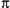home | O'Reilly's CD bookshelfs | FreeBSD | Linux | Cisco | Cisco Exam## 8.147. Math::Trig

Defines many trigonometric functions not defined by the core Perl, plus the constant pi and some additional functions for converting angles. For example:

```use Math::Trig;

\$x = tan(0.9);
\$halfpi = pi/2;```

The following functions are defined. A slash (/) between two functions means the values are aliases.

acsc/acosec, asec, acot/acotan
Arcus cofunctions of sine, cosine, and tangent

acsch/acosech, asech, acoth/acotanh
Arcus cofunctions of hyperbolic sine, cosine, and tangent

asin, acos, atan
Arcus (inverse) functions of sine, cosine, and tangent

asinh, acosh, atanh
Arcus functions of hyperbolic sine, cosine, and tangent

atan2(y, x)
Principal value of arc tangent of y/x

csc/cosec, sec, cot/cotan
Cofunctions of sine, cosine, and tangent

csch/cosech, sech, coth/cotanh
Cofunctions of hyperbolic sine, cosine, and tangent

pi
The trigonometric constant